# Configuring a DC Operating Point Analysis in Multisim

Publish Date: Mar 27, 2012 | 4 Ratings | 3.75 out of 5 | Print | Submit your review

## Overview

Multisim features a comprehensive suite of SPICE analyses for examining circuit behavior. These analyses range from the basic to sophisticated. Each analysis helps you to obtain valuable information such as the effects of component tolerances and sensitivities. For each analysis you need to set settings that will inform Multisim exactly what to analyze, and how.

Multisim simplifies the procedure for an advanced analysis by providing a configuration window. This abstracts away the complexities associated with SPICE syntax and configuration of an analysis. With this window you merely need to specify the parameter values and output nodes of interest.

This tutorial is part of the National Instruments SPICE Analysis Fundamentals Series. Each tutorial in this series provides you with step-by-step instructions on how to configure and run the different SPICE analyses available in Multisim.powerful simulation and analysis while abstracting the complexity of SPICE syntax.

### 1. Introduction

DC Operating Point Analysis calculates the behavior of a circuit when a DC voltage or current is applied to it. The result of this analysis is generally referred as the bias point or quiescent point, Q-point. In most cases, the results of the DC Operating Point Analysis are intermediate values for further analysis. For example, in AC Analysis, the DC operating point is first calculated to obtain linear, small-signal models for all nonlinear components (such a diodes and transistors).

Assumptions: AC sources are zeroed out, capacitors are open, inductors are shorted, digital components are treated as a large resistor to ground.

### 2. Running DC Operating Point Analysis

In this example, we calculate the DC value generated at the collector, VC, of the Colpitts oscillator circuit shown in Figure 1. You can get this file, colpitts_oscillator.ms11 from the Downloads section.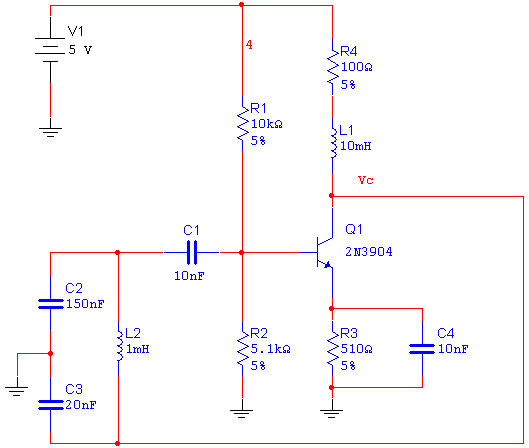Figure 1. Colpitts oscillator circuit.

Complete the following steps to configure and run a DC Operating Point Analysis.

1. Select Simulate»Analyses»DC Operating Point.

Note: In SPICE, the command that requests a DC operating point calculation is .OP. In this case there are no analysis parameters to be set, therefore the Analysis Parameters tab does not appear.

1. Select the Variables in circuit list, select All variables from the drop-down list, and then highlight V(vc) from the list.
1. Click the Add button to move the variable to the right side under Selected variables for analysis, as shown below.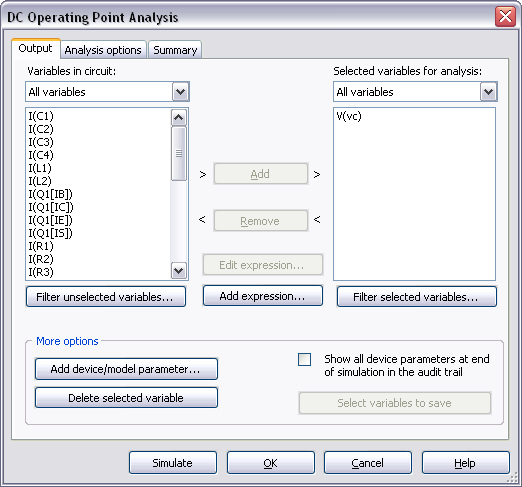Figure 2. Settings for the DC Operating Point Analysis.

1. Click Simulate to run the DC Operating Point Analysis. The Grapher View window opens and presents the result for V(vc):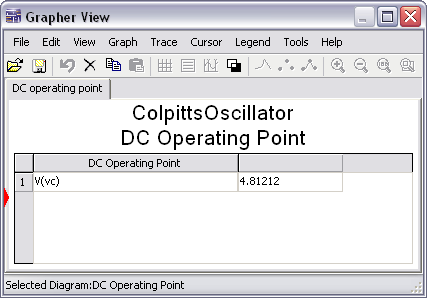Figure 3. Grapher View results for the DC Operating Point Analysis.

To verify this result you will analyze the Colpitts oscillator circuit shown in Figure 1. Keep in mind that when doing a DC Operating Point Analysis, inductors are shorted and capacitors are open. Figures 4a and 4b show the reduced circuit.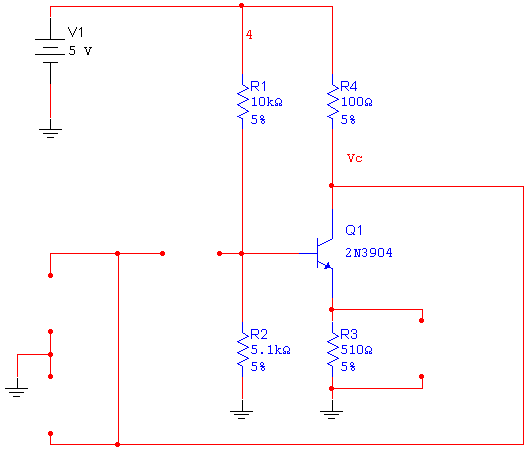(a)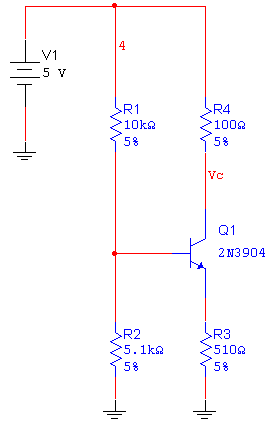(b)

Figure 4. Reduced circuit to calculate the DC operating point.

Use the following expressions to calculate the collector voltage, VC:

Divider current: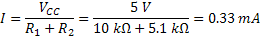Base voltage: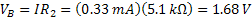Emitter voltage:Emitter current: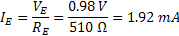Collector current: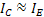Collector voltage: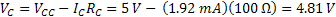Note that this calculation (VC = 4.81 V) matches the result obtained by the DC Operating Point Analysis in Multisim.

Since the DC Operating Point Analysis is often performed as the first step of other analyses, it is important to know how to solve convergence problems and analysis failures. Refer to the Troubleshooting DC Operating Point Analysis Failures article to learn more about it.

Entering Expressions in Analyses in Multisim

SPICE Analysis Fundamentals

Circuit Design Technical Library

Join the NI Circuit Design Community